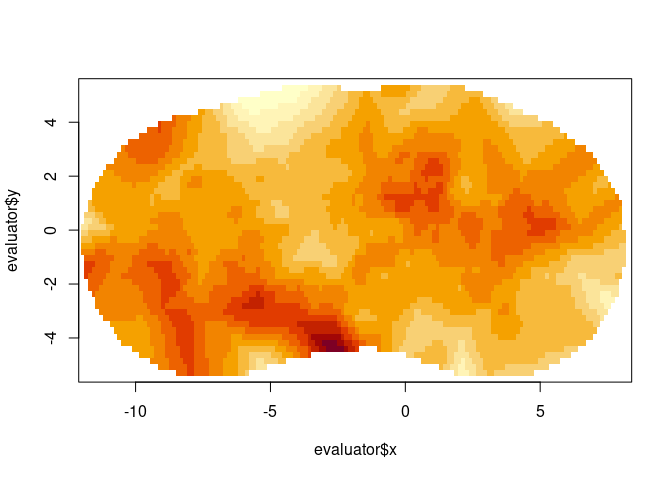# Basic fmesher use

suppressPackageStartupMessages(library(fmesher))
set.seed(1234L)

## Mesh construction

domain <- cbind(rnorm(4, sd = 3), rnorm(4))
(mesh2 <- fm_mesh_2d(
boundary = fm_extensions(domain, c(2.5, 5)),
max.edge = c(0.5, 2)
))
#> fm_mesh_2d object:
#>   Manifold:  R2
#>   V / E / T: 888 / 2622 / 1735
#>   Euler char.:   1
#>   Constraints:   39 boundary edges (1 group: 1), 115 interior edges (1 group: 1)
#>   Bounding box: (-11.963110,  8.246925) x (-5.522181, 5.499657) x (0,0)
#>   Basis d.o.f.:  888
plot(mesh2, axes = TRUE)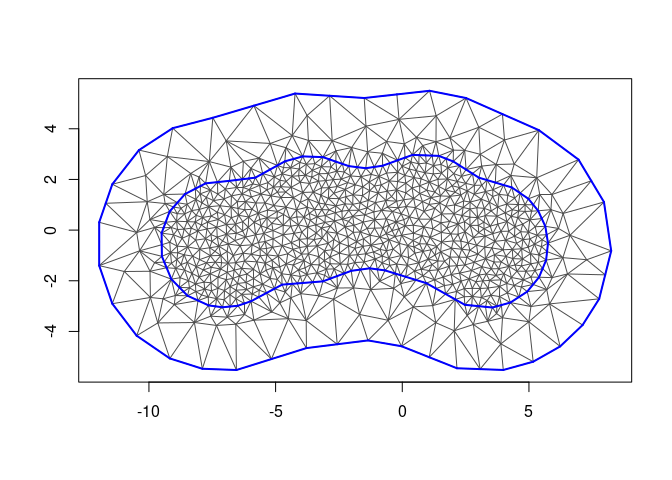(mesh1 <- fm_mesh_1d(
c(0, 2, 4, 7, 10),
boundary = "free", # c("neumann", "dirichlet"),
degree = 2
))
#> fm_mesh_1d object:
#>   Manifold:  R1
#>   #{knots}:  5
#>   Interval:  ( 0, 10)
#>   Boundary:  (free, free)
#>   B-spline degree:   2
#>   Basis d.o.f.:  6

## Point lookup and evaluation

pts <- cbind(rnorm(400, sd = 3), rnorm(400))

# Find what triangle each point is in, and it's triangular Barycentric coordinates
bary <- fm_bary(mesh2, loc = pts)
# How many points are outside the mesh?
sum(is.na(bary$t)) #>  2 head(bary$bary)
#>            [,1]      [,2]      [,3]
#> [1,] 0.04720217 0.5813611 0.3714367
#> [2,] 0.13950532 0.7212692 0.1392255
#> [3,] 0.43189448 0.1384910 0.4296145
#> [4,] 0.20031702 0.3721526 0.4275303
#> [5,] 0.21259133 0.3686084 0.4188003
#> [6,] 0.44390951 0.1739205 0.3821700

# Construct an evaluator object
evaluator <- fm_evaluator(mesh2, loc = pts)
sum(!evaluator$proj$ok)
#>  2

# Values for the basis function weights; for ordinary 2d meshes this coincides
# with the resulting values at the vertices, but this is not true for e.g.
# 2nd order B-splines on 1d meshes.
field <- mesh2$loc[, 1] value <- fm_evaluate(evaluator, field = field) sum(abs(pts[, 1] - value), na.rm = TRUE) #>  5.704812e-14 pts1 <- seq(-2, 12, length.out = 1000) # Find what segment, and its interval Barycentric coordinates bary1 <- fm_bary(mesh1, loc = pts1) # Points outside the interval are treated differently depending on the # boundary conditions: sum(is.na(bary1$t))
#>  0
head(bary1$bary) #> [,1] [,2] #> [1,] 2.000000 -1.000000 #> [2,] 1.992993 -0.992993 #> [3,] 1.985986 -0.985986 #> [4,] 1.978979 -0.978979 #> [5,] 1.971972 -0.971972 #> [6,] 1.964965 -0.964965 # Construct an evaluator object. evaluator1 <- fm_evaluator(mesh1, loc = pts1) # mesh_1d basis functions are defined everywhere sum(!evaluator1$proj$ok) #>  0 # Values for the basis function weights; for ordinary 2d meshes this coincides # with the resulting values at the vertices, but this is not true for e.g. # 2nd order B-splines on 1d meshes. field1 <- rnorm(fm_dof(mesh1)) value1 <- fm_evaluate(evaluator1, field = field1) plot(pts1, value1, type = "l")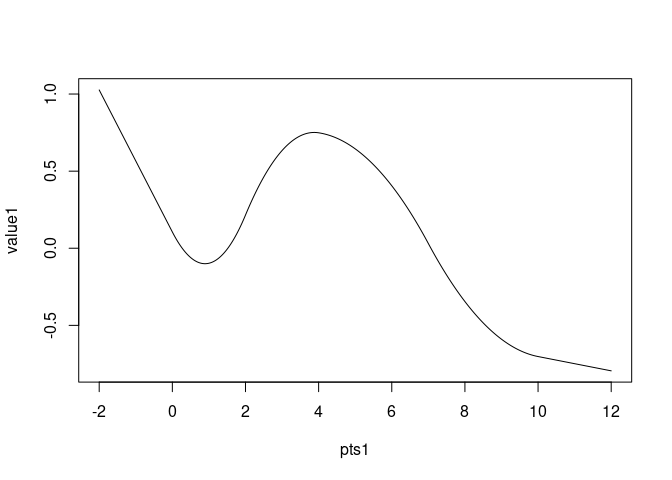## Plotting ### Base graphics plot(mesh2)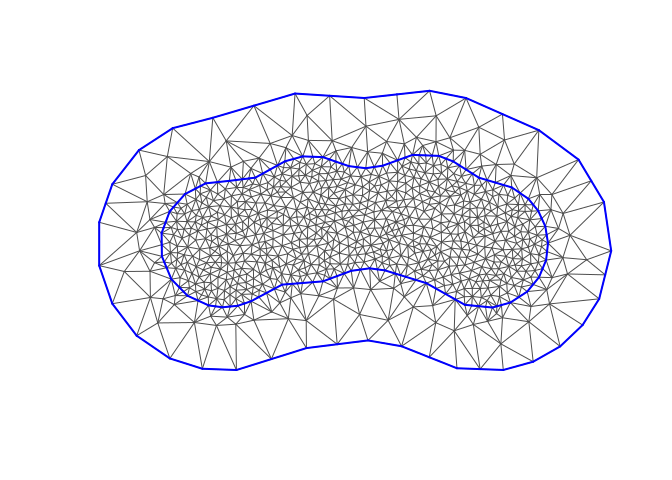### ggplot graphics suppressPackageStartupMessages(library(ggplot2)) ggplot() + geom_fm(data = mesh2)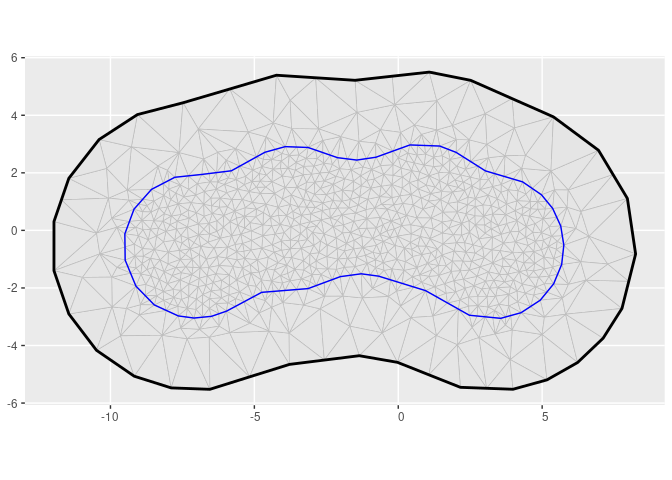ggplot() + geom_fm(data = mesh1, weights = field1 + 2, xlim = c(-2, 12)) + geom_fm(data = mesh1, linetype = 2, alpha = 0.5, xlim = c(-2, 12))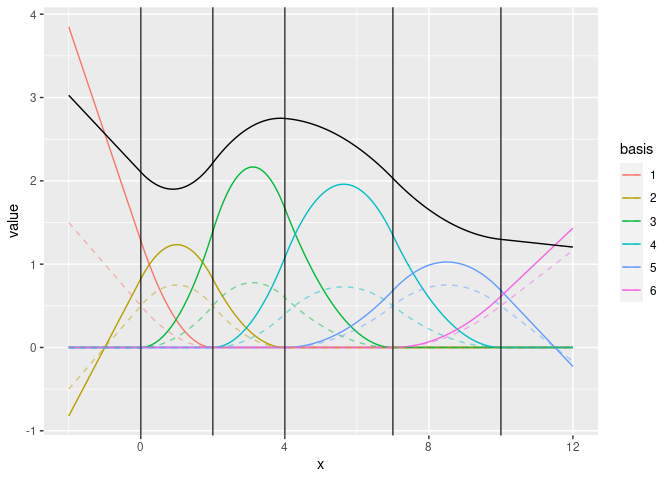## Finite element calculations fem1 <- fm_fem(mesh1, order = 2) names(fem1) #>  "c0" "c1" "g1" "g2" "g01" "g02" "g12" fem2 <- fm_fem(mesh2, order = 2) names(fem2) #>  "b1" "c0" "c1" "g1" "g2" "k1" "k2" "ta" "va" ## Stochastic process simulation samp <- fm_matern_sample(mesh2, alpha = 2, rho = 4, sigma = 1)[, 1] evaluator <- fm_evaluator(mesh2, lattice = fm_evaluator_lattice(mesh2, dims = c(150, 50))) image(evaluator$x, evaluator\$y, fm_evaluate(evaluator, field = samp), asp = 1)